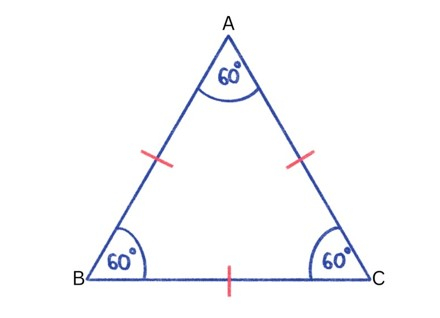# Swift Program to Calculate Area and Perimeter of Equilateral Triangle

This tutorial will discuss how to write swift program to calculate area and perimeter of equilateral triangle

A triangle whose all sides and angles are equal is known as the equilateral triangle. Each angle of equilateral triangle is 60 degrees. It is also known as 3-side polygon.## Area of Equilateral Triangle

The amount of space occupied between the three lines of the triangle is known as the area of the equilateral triangle.

### Formula

Following is the formula −

Area = √3x2/ 4


Here x is the side of the equilateral triangle.

Below is a demonstration of the same −

Input

Suppose our given input is −

Side = 4


Output

The desired output would be −

Area of the equilateral triangle: 6.928203230275509


### Algorithm

Following is the algorithm −

Step 1 - Create a function with return value.

Step 2 - Calculate the area of the equilateral triangle using the following formula −

let result = (sqrt(3) * side * side)/4


Step 3 - Calling the function and pass the side in the function as a parameter.

Step 4 - Print the output.

### Example

The following program shows how to calculate area of the equilateral triangle.

import Foundation
import Glibc

// Creating a function to calculate area of the equilateral triangle
func equiTriangle(side: Double){
let result = (sqrt(3) * side * side)/4
print("Area of the equilateral triangle: \(result)")
}

// Side of the equilateral triangle
var x = 10.0
print("Side: \(x)")

// Calling function
equiTriangle(side:x)


### Output

Side: 10.0
Area of the equilateral triangle: 43.301270189221924


Here, in the above program we create a function which return area of the equilateral triangle using the following formula −

let result = (sqrt(3) * side * side)/4


Here we enter side = 10, hence the area is 43.301270189221924.

## Perimeter of Equilateral Triangle

The distance around the edges of the equilateral triangle is known as the perimeter of the equilateral triangle.

### Formula

Following is the formula −

Perimeter = 3x


Here x is the side of the equilateral triangle.

Below is a demonstration of the same −

Input

Suppose our given input is −

Side = 7


Output

The desired output would be -

Perimeter of the equilateral triangle: 21


### Algorithm

Following is the algorithm −

Step 1 - Create a function with return value.

Step 2 - Calculate perimeter of the equilateral triangle using the following formula −

let result = 3 * side


Step 3 - Calling the function and pass the side in the function as a parameter.

Step 4 - Print the output.

### Example

The following program shows how to calculate perimeter of the equilateral triangle.

import Foundation
import Glibc

// Creating a function to calculate the perimeter

// of the equilateral triangle.
func periTriangle(side: Int){
let result = 3 * side
print("Perimeter of the equilateral triangle: \(result)")
}

// Side of the equilateral triangle
var x = 4
print("Side: \(x)")

// Calling function
periTriangle(side:x)


### Output

Side: 4
Perimeter of the equilateral triangle: 12


Here, in the above program we create a function which return the perimeter of the equilateral triangle using the following formula −

let result = 3 * side


Here we enter side = 4, hence the mid-point is 12.